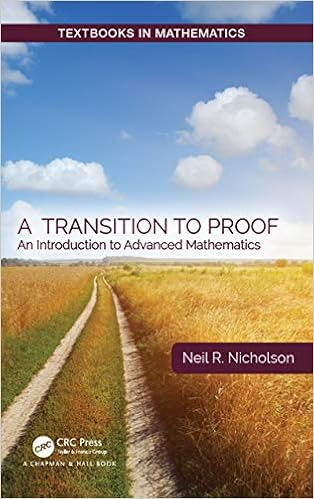# A Transition to Proof: An Introduction to Advanced Mathematics (Textbooks in Mathematics)

#### Tag: Mathematics

Posted on 2020-05-22, by freebook77.

DescriptionA Transition to Proof: An Introduction to Advanced Mathematics describes writing proofs as a creative process. There is a lot that goes into creating a mathematical proof before writing it. Ample discussion of how to figure out the "nuts and bolts'" of the proof takes place: thought processes, scratch work and ways to attack problems. Readers will learn not just how to write mathematics but also how to do mathematics. They will then learn to communicate mathematics effectively.

The text emphasizes the creativity, intuition, and correct mathematical exposition as it prepares students for courses beyond the calculus sequence. The author urges readers to work to define their mathematical voices. This is done with style tips and strict "mathematical do’s and don’ts", which are presented in eye-catching "text-boxes" throughout the text. The end result enables readers to fully understand the fundamentals of proof.

Features:

The text is aimed at transition courses preparing students to take analysis

Promotes creativity, intuition, and accuracy in exposition

The language of proof is established in the first two chapters, which cover logic and set theory

Includes chapters on cardinality and introductory topology

https://nitroflare.com/view/9251D5490A9A747/B07Q11CF25.pdf

7108 dl's @ 2416 KB/s
6755 dl's @ 2423 KB/s
8517 dl's @ 3510 KB/s

Search More...
A Transition to Proof: An Introduction to Advanced Mathematics (Textbooks in Mathematics)Related Books